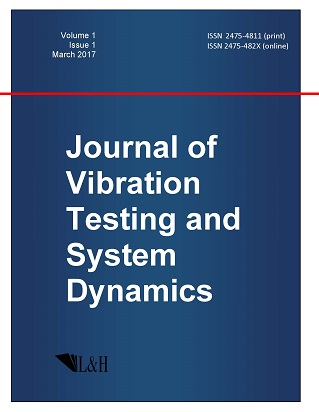ISSN: 2475-4811 (print)
ISSN: 2475-482X (online)
Journal of Vibration Testing and System Dynamics

C. Steve Suh (editor), Pawel Olejnik (editor),

Xianguo Tuo (editor)

Pawel Olejnik (editor)

Lodz University of Technology, Poland

Email: pawel.olejnik@p.lodz.pl

C. Steve Suh (editor)

Texas A&M University, USA

Email: ssuh@tamu.edu

Xiangguo Tuo (editor)

Sichuan University of Science and Engineering, China

Email: tuoxianguo@suse.edu.cn

Stability of Higher Order Nonlinear Implicit Fractional Differential Equations by Fixed Point Technique

Journal of Vibration Testing and System Dynamics 5(2) (2021) 169--180 | DOI:10.5890/JVTSD.2021.06.005

Kausika Chellamuthu

Department of Mathematics, Bharathiar University, Coimbatore, TN 641046, India

Abstract

This paper constructs a theoretical framework to analyze stability of higher order nonlinear implicit fractional differential equations. The main theorem asserts the stability and asymptotic stability by making use of Krasnoselskii's fixed point technique. Results are established in a weighted Banach space to arrive at the compactness requirements directly. Two examples are provided to illustrate the theory.

Acknowledgments

\noindent The author would like to thank Prof. Dr. K. Balachandran and Dr. N. Annapoorani, for their support and suggestions to carry out this research work.

References

1.   Burton, T.A. (1985), { Stability and Periodic Solutions of Ordinary and Functional Differential Equations}, Academic press, INC, Orlando, Florida.
2.   Burton, T.A. and Furumochi, T. (2001), A note on stability by Schauders theorem, { Funkcialaj Ekvacioj}, {\bf 44}, 73-82.
3.   Burton, T.A. (2006), The case for stability by fixed point theory, { Dynamics of Continuous, Discrete and Impulsive Systems Series A: Mathematical Analysis}, {\bf 13B}, 253-263.
4.   Balachandran, K. and Kokila, J. (2014), Controllability of non-linear implicit fractional dynamical systems, { Journal of Applied Mathematics}, {\bf 79}, 562-570.
5.   Balachandran, K., Park, J.Y., and Trujillo, J.J. (2012), Controllability of nonlinear fractional dynamical systems, { Nonlinear Analysis: Theory, Methods $\&$ Applications }, {\bf 75}, 1919-1926.
6.   Balachandran, K., Govindaraj, V., Rodriguez-Germ\{a}, L., and Trujillo, J.J. (2014), Stabilizability of fractional dynamical systems, { Fractional Calculus $\&$ Applied Analysis}, {\bf 17}, 511-531.
7.   Suresh Kumar, P., Balachandran, K., and Annapoorani, N. (2018), Controllability of nonlinear fractional Langevin delay systems, { Nonlinear Analysis-Modelling and Control}, {\bf 23}, 321-340.
8.   Qian, D., Li, C., Agarwal, R.P., and Wong, R.P. (2010), Stability analysis of fractional differential system with Riemann-Lioville derivative, { Mathematical and Computer Modelling}, {\bf 52}, 862-874.
9.   Li, Y., Chen, Y.Q., and Podlubny, I. (2010), Stability of fractional-order nonlinear dynamic systems: Lyapunov direct method and generalized Mittag-Leffler stability, { Computers $\&$ Mathematics with Applications}, {\bf 59}, 1810-1821.
10.   Fe\v{c}kan, M. and Wang, J.R. (2017), Mixed order fractional differential equations, { Mathematics}, {\bf 5}(61), 9 pages.
11.   Abbas, S., Benchohra, M., Graef, J., and Henderson, J. (2018), { Implicit Fractional Differential and Integral Equations; Existence and Stability}, De Gruyter, Berlin.
12.   Ardjouni, A., Boulares, H., and Laskri, Y. (2018), Stability in higher-order nonlinear fractional differential equations, { Acta Et Commentationes Universitatis Tartuensis De Mathematica}, {\bf 22}, 37-47.
13.   Ali, A., Shah, K., and Abdeljawad, T. (2020), Study of implicit delay fractional differential equations under anti-periodic boundary conditions, { Advances in Difference Equations}, {\bf 2020}, 139.
14.   Burton, T.A. (2006), { Stability by Fixed Point Theory for Functional Differential Equations}, Dover Publications, INC, Mineola, New York.
15.   Becker, L.C. (1979), { Stability considerations for Volterra integro-differential equations} (Ph.D. dissertation), Southern Illionis University.
16.   Burton, T.A. and Zhang, B. (2012), Fractional differential equations and generalizations of Schaefers and Krasnoselskiis fixed point theorems, { Nonlinear Analysis}, {\bf 75}, 6485-6495.
17.   Ge, F. and Kou, C. (2015), Stability analysis by Krasnoselskiis fixed point theorem for nonlinear fractional differential equations, { Applied Mathematics $\&$ Computation}, {\bf 257}, 308-316.
18.   Diethelm, K. (2010), { The Analysis of Fractional Differential Equations}, Springer-Verlag, Berlin.
19.   Kou, C., Zhou, H., and Yan, Y. (2011), Existence of solutions of initial value problems for nonlinear fractional differential equations on the half-axis, { Nonlinear Analysis}, {\bf 74}, 5975-5986.
20.   Krasnoselskii, M.A. (1958), Some problems of nonlinear analysis, { American Mathematical Society Translations}, {\bf 10}, 345-409.